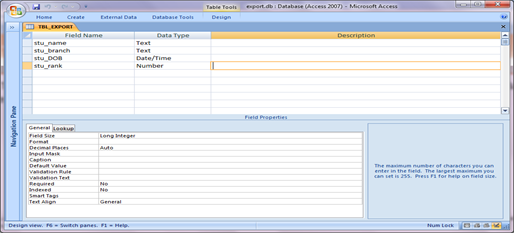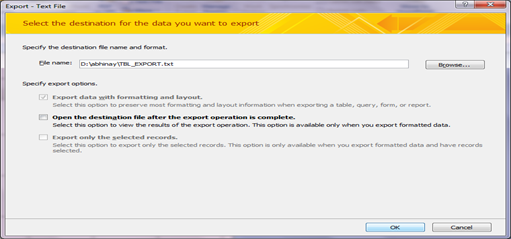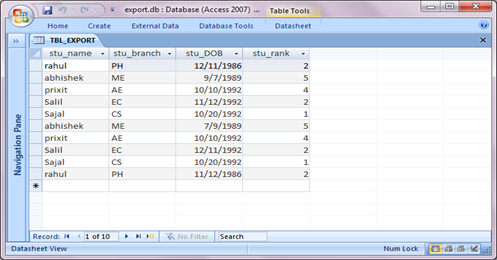# Export and import in Microsoft Access 2010 using VBA

Export and Import data into text format using VBA code.

This article is about to export table data to text file using VBA code and convert the data into text form. And this converted data in text file also import to table with original data form.

Firstly we are creating a table and save data that we want to convert into text file as shown in Fig 1.1.Fig:-1.1

Then we create a form and insert two buttons for exporting and importing. On the buttons event we have to call a function that import and export as shown in Fig 1.2Fig:-1.2

After the coding we have to set the layout, name and path where the text file is created is stored as shown in Fig 1.3. When we click on ok button a blank text file is created on the select destination.Fig:-1.3

Now when we have to open the form and click on export button. This will export data of table into text file as shown in Fig 1.4.Fig:-1.4

After the exporting the date we have to import text file data into table .For this we have to click on import button this will save copy the text data into original data on same table or we can change the table also as requirement as shown in Fig 1.5Fig:-1.5

VBA Code for import and export :

Option Compare Database

Private Sub cmdBtn_Click()
EXPORT "TBL_EXPORT"
End Sub

Private Sub cmdBtn2_Click()
IMPORT "TBL_EXPORT"
End Sub

' EXPORT FROM TABLE TO TEXT FILE
Public Function EXPORT(ByVal tblName As String)

Dim db As Database
Dim rst As Recordset
Dim nsize As Integer
Dim dtSize As Integer
Dim fld As Field, fldCount As Integer
Dim a As Integer
Dim tbldef As TableDef
Dim fmt As String
Dim outTxt As String
Dim outFileName As String
Dim tbsize() As Variant

On Error GoTo EXPORT_Err
dtSize = 10
nsize = 12
outFileName = tblName & ".txt"
Set db = CurrentDb
Set tbldef = db.TableDefs(tblName)
fldCount = tbldef.Fields.Count - 1
ReDim tbsize(fldCount)
For a = 0 To fldCount
Set fld = tbldef.Fields(a)
Select Case fld.Type
Case 3, 4, 6, 7, 20
tbsize(a) = String(nsize, "0")
Case 8
tbsize(a) = String(dtSize, "0")
Case 10
tbsize(a) = String(fld.Size, "x")
End Select
Next
Set rst = db.OpenRecordset(tblName)
Open outFileName For Output As #1
Do While Not rst.EOF
For a = 0 To fldCount
Set fld = tbldef.Fields(a)
Select Case fld.Type
Case 3, 4, 6, 7, 20
fmt = "00000000.000"
RSet tbsize(a) = Format(rst.Fields(a).Value, fmt)
Case 8
fmt = "dd/mm/yyyy"
RSet tbsize(a) = Format(rst.Fields(a).Value, fmt)
Case 10
LSet tbsize(a) = rst.Fields(a).Value
End Select
Next
outTxt = ""
For a = 0 To fldCount
outTxt = outTxt & tbsize(a)
Next
Print #1, outTxt
rst.MoveNext
Loop

Close #1
rst.Close
db.Close
Set db = Nothing
Set tbldef = Nothing
Set fld = Nothing
EXPORT_Exit:
Exit Function

EXPORT_Err:
MsgBox Err & " : " & Err.Description, , "EXPORT()"
Resume EXPORT_Exit
End Function

'IMPORT TO TEXT FILE TO TABLE
Public Function IMPORT(ByVal txtFileName As String)

Dim tbsize() As Variant
Dim db As Database, rst As Recordset
Dim fld As Field, fldCount As Integer
Dim a As Integer, tbldef As TableDef
Dim nsize As Integer, dtSize As Integer
Dim fmt As String, outTxt As String
Dim inputFileName As String
Dim I As Integer
Dim k As Integer

On Error GoTo IMPORT_Err
nsize = 12
dtSize = 10
inputFileName = txtFileName & ".txt"
Set db = CurrentDb
Set tbldef = db.TableDefs(txtFileName)
fldCount = tbldef.Fields.Count - 1
ReDim tbsize(fldCount)
For a = 0 To fldCount
Set fld = tbldef.Fields(a)
Select Case fld.Type
Case 3, 4, 6, 7, 20
tbsize(a) = String(nsize, "0")
Case 8
tbsize(a) = String(dtSize, "0")
Case 10
tbsize(a) = String(fld.Size, "x")
End Select
Next

Set rst = db.OpenRecordset(txtFileName)
Open inputFileName For Input As #1
Do While Not EOF(1)
Input #1, outTxt
I = 1
For a = 0 To fldCount
Set fld = tbldef.Fields(a)
k = Len(tbsize(a)
) Select Case fld.Type
Case 3, 4, 6, 7, 20
rst.Fields(a).Value = Val(Mid(outTxt, I, k))
I = I + k
Case 8
rst.Fields(a).Value = CDate(Mid(outTxt, I, k))
I = I + k
Case 10
rst.Fields(a).Value = Mid(outTxt, I, k)
I = I + k
End Select
Next

rst.Update
outTxt = ""
Loop

Close #1
rst.Close
db.Close
Set db = Nothing
Set tbldef = Nothing
Set fld = Nothing

IMPORT_Exit:
Exit Function

IMPORT_Err:
MsgBox Err & " : " & Err.Description, , "IMPORT()"
Resume IMPORT_Exit
End Function
End Function

DISCLAIMER

It is advised that the information provided in the article should not be used for any kind formal or production programming purposes as content of the article may not be complete or well tested. ERP Makers will not be responsible for any kind of damage (monetary, time, personal or any other type) which may take place because of the usage of the content in the article.